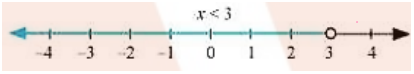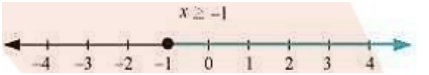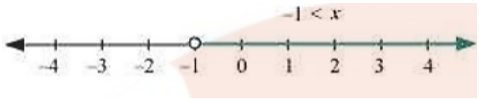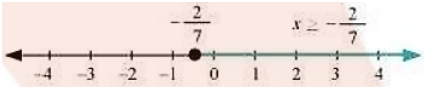# NCERT Class 11 Maths Linear Inequalities

## Miscellaneous Exercise

Download FREE PDF of Chapter-6 Linear Inequalities

## Chapter 6 Ex.6.1 Question 1

Solve $$24x \lt 100,$$ when

(i) $$x$$ is a natural number (ii) $$x$$ is an integer

### Solution

The given inequality is $$24x \lt 100,$$

$$24x \lt 100$$

$$\Rightarrow \frac{{24x}}{{24}} \lt \frac{{100}}{{24}}$$ [Dividing both sides by same positive number]

$$\Rightarrow x \lt \frac{{25}}{6}$$

(i) It is evident that $$1,2,3$$ and $$4$$ are the only natural numbers less than $$\frac{{25}}{6}$$

Thus, when $$x$$ is a natural number, the solutions of the given inequalities are $$1,2,3$$ and $$4$$

Hence, in this case, the solution set is$$\left\{ {1,2,3,4} \right\}$$ .

(ii) The integer less than $$\frac{{25}}{6}$$ are $$\ldots . - 3, - 2, - 1,0,1,2,3,4$$ .

Thus, when $$x$$ is an integer, the solutions of the given inequality are $$\ldots ,~-3,-2,-1,0,1,2,3,4$$

Hence, in this case, the solution set is$$\left\{ { - 3, - 2, - 1,0,1,2,3,4} \right\}$$

## Chapter 6 Ex.6.1 Question 2

Solve $$- 12x > 30$$ , when

(i) x is a natural number

(ii) x is an integer

### Solution

The given inequality is $$- 12x \gt 30$$

\begin{align} &\Rightarrow - 12x \gt 30 &\\ \ &\Rightarrow \frac{{ - 12x}}{{ - 12}} \lt \frac{{30}}{{ - 12}} \text{ [Dividing both sides by same negative number]} \\ &\Rightarrow x \lt - \frac{5}{2} \end{align}

(i) There is no natural number less than $$\left( { - \frac{5}{2}} \right)$$.

Thus, when x is a natural number, there is no solution of the given inequality.

(ii) The integer less than $$\left( { - \frac{5}{2}} \right)$$ are $$\ldots , - 5, - 4, - 3$$ .

Thus, when x is an integer, the solutions of the given inequality are $$\ldots , - 5, - 4, - 3$$

Hence, in this case, the solution set is $$\left\{ { \ldots , - 5, - 4, - 3} \right\}$$ .

## Chapter 6 Ex.6.1 Question 3

Solve $$5x - 3 \lt 7$$ , when

(i) $$x$$ is an integer

(ii) $$x$$ is a real number

### Solution

The given inequality is $$5x - 3 \lt 7$$.

\begin{align}\Rightarrow\quad&5x - 3 \lt 7\\ \Rightarrow\quad& 5x - 3 + 3 \lt 7 + 3\\ \Rightarrow\quad&5x \lt 10\\ \Rightarrow\quad&\frac{{5x}}{5} \lt \frac{{10}}{5}\\ \Rightarrow\quad&x \lt 2\end{align}

(i) The integers less than $$2$$ are $$\ldots , - 4, - 3, - 2, - 1,0,1$$ .

Thus, when $$x$$ is an integer, the solutions of the given inequality are $$\ldots , - 4, - 3, - 2, - 1,0,1$$

Hence, in this case, the solution set is $$\left\{ { \ldots , - 4, - 3, - 2, - 1,0,1} \right\}$$

(ii) When $$x$$ is a real number, the solutions of the given inequality are given by $$x \lt 2$$ that is, all real numbers $$x$$ which are less than $$2$$.

Thus, the solution set of the given inequality is $$\left( { - \infty ,2} \right)$$.

## Chapter 6 Ex.6.1 Question 4

Solve $$3x + 8 \gt 2$$, when

(i) $$x$$ is an integer

(ii) $$x$$ is a real number

### Solution

The given inequality is $$3x + 8 \gt 2$$

\begin{align}&3x + 8 \gt 2\\\Rightarrow\quad& 3x + 8 - 8 \gt 2 - 8\\\Rightarrow\quad& 3x \gt - 6\\\Rightarrow\quad& \frac{{3x}}{3} \gt \frac{{ - 6}}{3}\\\Rightarrow\quad& x \gt - 2\end{align}

(i) The integers greater than $$- 2$$ are $$- 1,0,1,2, \ldots$$

Thus, when x is an integer, the solutions of the given inequality are $$- 1,0,1,2 \ldots$$

Hence, in this case, the solution set is $$\left\{ -1,0,1,2,\ldots \right\}$$ .

(ii) When x is a real number, the solutions of the given inequality are all the real numbers, which are greater than$$-2$$.

Thus, in this case the solution set is $$\left( { - 2,\infty } \right)$$.

## Chapter 6 Ex.6.1 Question 5

Solve the given inequality for real $$x:4x + 3 \lt 5x + 7$$

### Solution

\begin{align}&4x + 3 \lt 5x + 7\\\Rightarrow\quad& 4x + 3 - 7 \lt 5x + 7 - 7\\\Rightarrow\quad&4x - 4 \lt 5x\\\Rightarrow\quad&4x - 4 - 4x \lt 5x - 4x\\\Rightarrow\quad&- 4 \lt x\\\Rightarrow\quad&x \gt - 4\end{align}

Thus, all real numbers $$x$$, which are greater than$$- 4$$ , are the solutions of the given inequality.

Hence, the solution set of the given inequality is $$\left( { - 4,\infty } \right)$$.

## Chapter 6 Ex.6.1 Question 6

Solve the given inequality for real $$x:3x - 7 \gt 5x - 1$$

### Solution

\begin{align}&3x - 7 \gt 5x - 1\\\Rightarrow\quad& 3x - 7 + 7 \gt 5x - 1 + 7\\\Rightarrow\quad& 3x \gt 5x + 6\\\Rightarrow\quad& 3x - 5x \gt 5x + 6 - 5x\\\Rightarrow\quad& - 2x \gt 6\\\Rightarrow\quad& - x \gt 3\\\Rightarrow\quad& x \lt - 3\end{align}

Thus, all real numbers $$x$$, which are less than $$- 3$$ , are the solutions of the given inequality. Hence, the solution set of the given inequality is $$\left( { - \infty , - 3} \right)$$

## Chapter 6 Ex.6.1 Question 7

Solve the given inequality for real $$x:3\left( {x - 1} \right) \le 2\left( {x - 3} \right)$$

### Solution

\begin{align}&3\left( {x - 1} \right) \le 2\left( {x - 3} \right)\\\Rightarrow\quad& 3x - 3 \le 2x - 6\\\Rightarrow\quad&3x - 3 + 3 \le 2x - 6 + 3\\\Rightarrow\quad&3x \le 2x - 3\\\Rightarrow\quad&3x - 2x \le 2x - 3 - 2x\\\Rightarrow\quad&x \le - 3\end{align}

Thus, all real numbers $$x$$, which are less than or equal to $$- 3$$ , are the solutions of the given inequality.

Hence, the solution set of the given inequality is $$\left( { - \infty , - 3} \right]$$

## Chapter 6 Ex.6.1 Question 8

Solve the given inequality for real $$x:3\left( {2 - x} \right) \ge 2\left( {1 - x} \right)$$

### Solution

\begin{align}&3\left( {2 - x} \right) \ge 2\left( {1 - x} \right)\\\Rightarrow\quad& 6 - 3x \ge 2 - 2x\\\Rightarrow\quad& 6 - 3x + 2x \ge 2 - 2x + 2x\\\Rightarrow\quad& 6 - x \ge 2\\\Rightarrow\quad& 6 - x - 6 \ge 2 - 6\\\Rightarrow\quad& - x \ge - 4\\\Rightarrow\quad& x \le 4\end{align}

Thus, all real numbers $$x$$, which are less than or equal to $$4$$, are the solutions of the given inequality.

Hence, the solution set of the given inequality is $$\left( { - \infty ,4} \right]$$

## Chapter 6 Ex.6.1 Question 9

Solve the given inequality for real $$x:x + \frac{x}{2} + \frac{x}{3} \lt 11$$

### Solution

\begin{align}&x + \frac{x}{2} + \frac{x}{3} \lt 11\\\Rightarrow\quad&x\left( {1 + \frac{1}{2} + \frac{1}{3}} \right) \lt 11\\\Rightarrow\quad&x\left( {\frac{{6 + 3 + 2}}{6}} \right) \lt 11\\\Rightarrow\quad&\frac{{11}}{6}x \lt 11\\\Rightarrow\quad&\frac{{11x}}{{6 \times 11}} \lt \frac{{11}}{{11}}\\\Rightarrow\quad&\frac{x}{6} \lt 1\\\Rightarrow\quad&x \lt 6\end{align}

Thus, all real numbers $$x$$, which are less than $$6$$, are the solutions of the given inequality.

Hence, the solution set of the given inequality is $$\left( { - \infty ,6} \right)$$.

## Chapter 6 Ex.6.1 Question 10

Solve the given inequality for real $$x:\frac{x}{3} \gt \frac{x}{2} + 1.$$

### Solution

\begin{align}&\frac{x}{3} \gt \frac{x}{2} + 1\\\Rightarrow\quad& \frac{x}{3} - \frac{x}{2} \gt 1\\\Rightarrow\quad&\frac{{2x - 3x}}{6} \gt 1\\\Rightarrow\quad&- \frac{x}{6} \gt 1\\\Rightarrow\quad&- x \gt 6\\\Rightarrow\quad&x \lt - 6\end{align}

Thus, all real numbers $$x$$, which are less than $$- 6$$ , are the solutions of the given inequality.

Hence, the solution set of the given inequality is $$\left( { - \infty , - 6} \right)$$

## Chapter 6 Ex.6.1 Question 11

Solve the given inequality for real $$x:\frac{{3\left( {x - 2} \right)}}{5} \le \frac{{5\left( {2 - x} \right)}}{3}$$

### Solution

\begin{align}&\frac{{3\left( {x - 2} \right)}}{5} \le \frac{{5\left( {2 - x} \right)}}{3}\\\Rightarrow\quad& 9\left( {x - 2} \right) \le 25\left( {2 - x} \right)\\\Rightarrow\quad& 9x - 18 \le 50 - 25x\\\Rightarrow\quad&9x + 25x \le 50 + 18\\\Rightarrow\quad&34x \le 68\\\Rightarrow\quad&x \le \frac{{68}}{{34}}\\\Rightarrow\quad&x \le 2\end{align}

Thus, all real numbers $$x$$, which are less than or equal to $$2$$, are the solutions of the given inequality.

Hence, the solution set of the given inequality is $$\left( { - \infty ,2} \right]$$

## Chapter 6 Ex.6.1 Question 12

Solve the given inequality for real $$x:\frac{1}{3}\left( {\frac{{3x}}{5} + 4} \right) \ge \frac{1}{3}\left( {x - 6} \right)$$

### Solution

\begin{align}&\frac{1}{2}\left( {\frac{{3x}}{5} + 4} \right) \ge \frac{1}{3}\left( {x - 6} \right)\\\Rightarrow\quad&3\left( {\frac{{3x}}{5} + 4} \right) \ge 2(x - 6)\\\Rightarrow\quad& \frac{{9x}}{5} + 12 \ge 2x - 12\\\Rightarrow\quad&12 + 12 \ge 2x - \frac{{9x}}{5}\\\Rightarrow\quad&24 \ge \frac{{10x - 9x}}{5}\\\Rightarrow\quad&24 \ge \frac{x}{5}\\\Rightarrow\quad&x \le 120\end{align}

Thus, all real numbers $$x$$, which are less than or equal to $$120$$, are the solutions of the given inequality.

Hence, the solution set of the given inequality is $$\left( { - \infty ,120} \right]$$

## Chapter 6 Ex.6.1 Question 13

Solve the given inequality for real $$x:2\left( {2x + 3} \right) - 10 \lt 6\left( {x - 2} \right)$$

### Solution

\begin{align}&2\left( {2x + 3} \right) - 10 \lt 6\left( {x - 2} \right)\\\Rightarrow\quad& 4x + 6 - 10 \lt 6x - 12\\\Rightarrow\quad&4x - 4 \lt 6x - 12\\\Rightarrow\quad&12 - 4 \lt 6x - 4x\\\Rightarrow\quad&8 \lt 2x\\\Rightarrow\quad&x \gt 4\end{align}

Thus, all real numbers $$x$$, which are greater than $$4$$, are the solutions of the given inequality.

Hence, the solution set of the given inequality is $$\left( {4,\infty } \right)$$ .

## Chapter 6 Ex.6.1 Question 14

Solve the given inequality for real $$x:37 - \left( {3x + 5} \right) \ge 9x - 8\left( {x - 3} \right)$$

### Solution

\begin{align}&37 - \left( {3x + 5} \right) \ge 9x - 8\left( {x - 3} \right)\\\Rightarrow\quad& 37 - 3x - 5 \ge 9x - 8x + 24\\\Rightarrow\quad&32 - 3x \ge x + 24\\\Rightarrow\quad&32 - 24 \ge x + 3x\\\Rightarrow\quad&8 \ge 4x\\\Rightarrow\quad&x \le 2\end{align}

Thus, all real numbers $$x$$, which are less than or equal to $$2$$, are the solutions of the given inequality.

Hence, the solution set of the given inequality is $$\left( { - \infty ,2} \right]$$

## Chapter 6 Ex.6.1 Question 15

Solve the given inequality for real $$x:\frac{x}{4} \lt \frac{{\left( {5x - 2} \right)}}{3} + \frac{{\left( {7x - 3} \right)}}{5}$$

### Solution

\begin{align}&\frac{x}{4} \lt \frac{{\left( {5x - 2} \right)}}{3} + \frac{{\left( {7x - 3} \right)}}{5}\\\Rightarrow\quad& \frac{x}{4} \lt \frac{{5(5x - 2) - 3(7x - 3)}}{{15}}\\\Rightarrow\quad& \frac{x}{4} \lt \frac{{25x - 10 - 21x + 9}}{3}\\\Rightarrow\quad& \frac{x}{4} \lt \frac{{4x - 1}}{{15}}\\\Rightarrow\quad& 15x \lt 4(4x - 1)\\\Rightarrow\quad& 15x \lt 16x - 4\\\Rightarrow\quad& 4 \lt 16x - 15x\\\Rightarrow\quad& x \gt 4\end{align}

Thus, all real numbers $$x$$, which are greater than $$4$$, are the solutions of the given inequality.

Hence, the solution set of the given inequality is $$\left( {4,\infty } \right)$$

## Chapter 6 Ex.6.1 Question 16

Solve the given inequality for real $$x:\frac{{\left( {2x - 1} \right)}}{3} \ge \frac{{\left( {3x - 2} \right)}}{4} - \frac{{\left( {2 - x} \right)}}{5}$$

### Solution

\begin{align}&\frac{{\left( {2x - 1} \right)}}{3} \ge \frac{{\left( {3x - 2} \right)}}{4} - \frac{{\left( {2 - x} \right)}}{5}\\\Rightarrow\quad& \frac{{\left( {2x - 1} \right)}}{3} \ge \frac{{5\left( {3x - 2} \right) - 4\left( {2 - x} \right)}}{{20}}\\\Rightarrow\quad& \frac{{\left( {2x - 1} \right)}}{3} \ge \frac{{15x - 10 - 8 + 4x}}{{20}}\\\Rightarrow\quad& \frac{{(2x - 1)}}{3} \ge \frac{{19x - 18}}{{20}}\\\Rightarrow\quad& 20\left( {2x - 1} \right) \ge 3\left( {19x - 18} \right)\\\Rightarrow\quad& 40x - 20 \ge 57x - 54\\\Rightarrow\quad& 40x - 57x \ge 20 - 54\\\Rightarrow\quad& - 17x \ge - 34\\\Rightarrow\quad& x \le 2\end{align}

Thus, all real numbers $$x$$, which are less than or equal to $$2$$, are the solutions of the given inequality.

Hence, the solution set of the given inequality is $$\left( { - \infty ,2} \right]$$

## Chapter 6 Ex.6.1 Question 17

Solve the given inequality and show the graph of the solution on number line:

$$3x - 2 \lt 2x + 1$$

### Solution

\begin{align}&3x - 2 \lt 2x + 1\\\Rightarrow\quad& 3x - 2x \lt 1 + 2\\\Rightarrow\quad&x \lt 3\end{align}

Hence, the solution set of the given inequality is $$\left( { - \infty ,3} \right)$$

The graphical representation of the solutions of the given inequality is as follows:## Chapter 6 Ex.6.1 Question 18

Solve the given inequality and show the graph of the solution on number line:

$$5x - 3 \ge 3x - 5$$

### Solution

\begin{align}&5x - 3 \ge 3x - 5\\\Rightarrow\quad& 5x - 3x \ge - 5 + 3\\\Rightarrow\quad& 2x \ge - 2\\\Rightarrow\quad& x \ge - 1\end{align}

Hence, the solution set of the given inequality is $$\left[ { - 1,\infty } \right)$$

The graphical representation of the solutions of the given inequality is as follows:## Chapter 6 Ex.6.1 Question 19

Solve the given inequality and show the graph of the solution on number line: $$3\left( {1 - x} \right) \lt 2\left( {x + 4} \right)$$

### Solution

\begin{align}&3\left( {1 - x} \right) \lt 2\left( {x + 4} \right)\\\Rightarrow\quad& 3 - 3x \lt 2x + 8\\\Rightarrow\quad& 3 - 8 \lt 2x + 3x\\\Rightarrow\quad& - 5 \lt 5x\\\Rightarrow\quad& x \gt - 1\end{align}

Hence, the solution set of the given inequality is $$\left( { - 1,\infty } \right)$$

The graphical representation of the solution of the inequality is as follows:## Chapter 6 Ex.6.1 Question 20

Solve the given inequality and show the graph of the solution on number line:

$$\frac{x}{2} \ge \frac{{\left( {5x - 2} \right)}}{3} - \frac{{\left( {7x - 3} \right)}}{5}$$

### Solution

\begin{align}&\frac{x}{2} \ge \frac{{\left( {5x - 2} \right)}}{3} - \frac{{\left( {7x - 3} \right)}}{5}\\ \Rightarrow\quad& \frac{x}{2} \ge \frac{{5(5x - 2) - 3(7x - 2)}}{{15}} \\ \Rightarrow\quad& \frac{x}{2} \ge \frac{{25x - 10 - 21x + 9}}{{15}}\\\Rightarrow\quad& \frac{x}{2} \ge \frac{{4x - 1}}{{15}}\\\Rightarrow\quad& 15x \ge 8x - 2\\\Rightarrow\quad& 15x - 8x \ge - 2\\\Rightarrow\quad& 7x \ge - 2\\\Rightarrow\quad& x \ge - \frac{2}{7}\end{align}

Hence, the solution set of the given inequality is $$\left[ { - \frac{2}{7},\infty } \right)$$

The graphical representation of the solution of the given inequality is as follows:## Chapter 6 Ex.6.1 Question 21

Ravi obtained $$70$$ and $$75$$ marks in first two-unit test. Find the minimum marks he should get in the third test to have an average of at least $$60$$ marks.

### Solution

Let $$x$$ be the marks obtained by Ravi in the third unit test.

Since the students should have an average of at least 60 marks,

\begin{align}&\frac{{70 + 75 + x}}{3} \ge 60\\\Rightarrow\quad&145 + x \ge 180\\\Rightarrow\quad& x \ge 180 - 145\\\Rightarrow\quad & x \ge 35\end{align}

Thus, the student must obtain a minimum of $$35$$ marks to have an average of at least 60 marks.

## Chapter 6 Ex.6.1 Question 22

To receive Grade ‘A’ in a course, one must obtain an average of $$90$$ marks or more in five examinations (each of $$100$$ marks). If Sunita’s marks in first four examinations are $$87, 92, 94$$ and $$95$$, find minimum marks that Sunita must obtain in fifth examination to get grade ‘A’ in the course.

### Solution

Let x be the marks obtained by Sunita in the fifth examination.

In order to receive grade ‘A’ in the course, she must obtain an average of $$90$$ marks or more in five examinations.

Therefore,

\begin{align}&\frac{{87 + 92 + 94 + 95 + x}}{5} \ge 90\\&\Rightarrow\quad \frac{{368 + x}}{5} \ge 90\\&\Rightarrow\quad 368 + x \ge 450\\&\Rightarrow\quad x \ge 450 - 368\\&\Rightarrow \quad x \ge 82\end{align}

Thus, Sunita must obtain a minimum of $$82$$ marks in the fifth examination

## Chapter 6 Ex.6.1 Question 23

Find all pairs of consecutive odd positive integers both of which are smaller than $$10$$ such that their sum is more than $$11$$.

### Solution

Let $$x$$ be the smaller of the two consecutive odd positive integers. Then, the other integer is $$\left( {x + 2} \right)$$

Since both the integers are smaller than $$10$$,

\begin{align}&\quad x + 2 \lt 10\\&\Rightarrow\quad x \lt 10 - 2\\&\Rightarrow\quad x \lt 8\;\;\;\; \quad\;\qquad \ldots \left( 1 \right)\end{align}

Also, the sum of the two integers is more than $$11$$.

Therefore,

\begin{align}&\quad x + \left( {x + 2} \right) \gt 11\\&\quad \Rightarrow\quad 2x + x \gt 11\\&\quad\Rightarrow\quad 2x \gt 11 - 2\\&\quad\Rightarrow\quad 2x \gt 9\\&\quad\Rightarrow\quad x \gt \frac{9}{2}\\&\quad\Rightarrow\quad x \gt 4.5\quad\qquad \ldots \left( 2 \right)\end{align}

From ($$1$$) and ($$2$$), we obtain

$4.5 \lt x \lt 8$

Since $$x$$ is an odd positive integer, then values of $$x$$ are $$5$$ and $$7$$.

When $$x = 5$$, the pair is $$\left( {5,7} \right)$$ and when $$x = 7$$, the pair is $$\left( {7,9} \right)$$

Thus, the required possible pairs are $$\left( {5,7} \right)$$ and $$\left( {7,9} \right)$$.

## Chapter 6 Ex.6.1 Question 24

Find all pairs of consecutive even positive integers, both of which are larger than  $$5$$ such that their sum is less than $$23$$.

### Solution

Let $$x$$ be the smaller of the two consecutive even positive integers, then the other integer is $$\left( {x + 2} \right)$$

Since both the integers are larger than $$5$$,

\begin{align}\qquad x \gt 5\;\;\;\;\;\;\;\;\;\;\;\;\;\;\;\;\;\; \ldots \left( 1 \right)\end{align}

Also, the sum of the two integers is less than $$23$$

\begin{align} &x + \left( {x + 2} \right) \lt 23 \\& \Rightarrow 2x + 2 \lt 23 \\& \Rightarrow 2x \lt 21 \\& \Rightarrow x \lt \frac{{21}}{2} \\& \Rightarrow x \lt 10.5\quad\;\;\;\;\qquad \ldots \left( 2 \right)\end{align}

From ($$1$$) and ($$2$$), we obtain

$$\qquad\qquad\qquad\qquad\qquad\qquad\qquad 5 \lt x \lt 10.5$$

Since $$x$$ is an even positive integer, then values of $$x$$ are $$6, 8$$ and $$10$$.

When $$x = 6$$, the pair is $$\left( {6,8} \right)$$

When $$x = 8$$, the pair is $$\left( {8,10} \right)$$

When $$x = 10$$, the pair is $$\left( {10,12} \right)$$

Thus, the required possible pairs are $$\left( 6,8 \right)$$, $$\left( 8,10 \right)$$ and $$\left( 10,12 \right)$$.

## Chapter 6 Ex.6.1 Question 25

The longest side of a triangle is $$3$$ times the shortest side and the third side is $$2$$ cm shorter than the longest side. If the perimeter of the triangle is at least $$61$$ cm,find the minimum length of the shortest side.

### Solution

Let the length of the shortest side of the triangle in cm be $$x$$.

Then, length of the longest side in cm $$= 3x$$

Length of the third side in cm $$= \left( {3x - 2} \right)$$

Since the perimeter of the triangle is at least $$61$$ cm,

Therefore,

\begin{align}&x + 3x + \left( {3x - 2} \right) \ge 61\\&\Rightarrow\quad 7x - 2 \ge 61\\&\Rightarrow\quad 7x \ge 61 + 2\\&\Rightarrow\quad 7x \ge 63\\&\Rightarrow\quad x \ge \frac{{63}}{7}\\&\Rightarrow\quad x \ge 9\end{align}

Thus, the minimum length of the shortest side is $$9$$ cm.

## Chapter 6 Ex.6.1 Question 26

A man wants to cut three lengths from a single piece of board of length $$91$$ cm. The second length is to be $$3$$ cm longer than the shortest and the third length is to be twice as long as the shortest. What are the possible lengths of the shortest board if the third piece is to be at least $$5$$ cm longer than the second?

[Hint: If x is the length of the shortest board, then $$\left( {x + 3} \right)$$ and $$2x$$ are the length of the second and third piece, respectively. Thus $$x = \left( {x + 3} \right) + 2x \le 91$$ and $$2x \ge \left( {x + 3} \right) + 5$$]

### Solution

Let the length of the shortest piece in cm be $$x$$.

Then, the length of second and third piece in cm are $$\left( {x + 3} \right)$$ and $$2x$$ respectively.

Since the three lengths are to be cut from a single piece of board of length $$91$$ cm.

\begin{align}&x + \left( {x + 3} \right) + 2x \le 91\\&\Rightarrow\quad 4x + 3 \le 91\\&\Rightarrow\quad 4x \le 91 - 3\\&\Rightarrow\quad 4x \le 88\\&\Rightarrow\quad x \le \frac{{88}}{4}\\&\Rightarrow\quad x \le 22\;\;\;\;\;\; \ldots \left( 1 \right)\end{align}

Also, the third piece is at least $$5$$ cm longer than the second piece.

\begin{align}& 2x \ge \left( {x + 3} \right) + 5\\&\Rightarrow\quad 2x \ge x + 8\\&\Rightarrow\quad 2x - x \ge 8\\&\Rightarrow\quad x \ge 8\qquad\;\; \ldots \left( 2 \right)\end{align}

From ($$1$$) and ($$2$$), we obtain

$8 \le x \le 22$

Thus, the possible length of the shortest board is greater than or equal to $$8$$ cm but less than or equal to $$22$$ cm.

Download FREE PDF of Chapter-6 Linear Inequalities
Linear Inequalities | NCERT Solutions
Learn from the best math teachers and top your exams

• Live one on one classroom and doubt clearing
• Practice worksheets in and after class for conceptual clarity
• Personalized curriculum to keep up with school